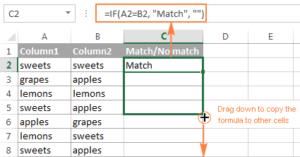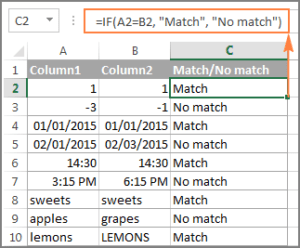# Compare 2 Consecutive Columns in Excel Row by Row

When performing data analysis in Excel, one of the most frequent tasks is comparing data in each individual row. This task can be done by using the IF function.

### Compare two columns for matches or differences in the same row

To compare two columns in Excel row-by-row, write a usual IF formula that compares the first two cells. Enter the formula in some other column in the same row, and then copy it down to other cells by dragging the fill handle (a small square in the bottom-right corner of the selected cell). As you do this, the cursor changes to the plus sign:### Formula for matches

To find cells within the same row having the same content, A2 and B2 in this example, the formula is as follows:

=IF(A2=B2,”Match”,””)

### Formula for differences

To find cells in the same row with different content, simply replace “=” with the non-equality sign:

=IF(A2<>B2,”No match”,””)

### Matches and differences

And of course, nothing prevents you from finding both matches and differences with a single formula:

=IF(A2=B2,”Match”,”No match”)

Or

=IF(A2<>B2,”No match”,”Match”)

The result may look similar to this:As you see, the formula works well with dates, numbers, test and time.